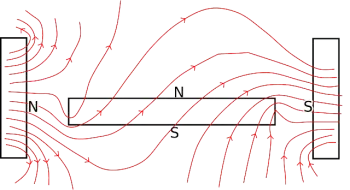# What Is a Magnetic Field? Examples and DefinitionA magnetic field is a field of force produced by moving electrical charges. This force field is applied on other non-parallel moving loads. Together with the electric field, the magnetic field constitutes the electromagnetic field, responsible for the electromagnetic interaction in space.

From the point of view of magnetism, materials can be classified into:

• Paramagnetic materials
• Diamagnetic materials
• Ferromagnetic materials
• Ferrimagnetic materials
• Antiferromagnetic materials
• This depends on the behavior of your magnetic susceptibility.

## What Is the Unit of Measurement for the Magnetic Field?

The unit of magnetic field in the International System of Units is the tesla (T). A tesla represents the field that generates a force of 1 newton (N) on an electric charge of 1 coulomb (C) moving within the field and perpendicular to the direction of the magnetic field lines at a speed of 1 m / s.

However, because the tesla is a very large unit, the gauss (G) is sometimes used: 10,000 G equals 1 T.

## Characteristics of a Magnetic Field

The magnetic field can be approached in a similar way to the electric one, but instead of considering the electric charge (a scalar) as the source of the field, this paper will be the magnetic dipole moment (a vector).

Magnetic forces are proportional to the generated magnetic field, that is, to the value of the magnetic induction which is a vector quantity used to characterize a field.

The relationship between the magnetic field and an electric current is given by Ampère's law. The most general case, which includes the displacement current, is given by the Ampère-Maxwell law.

## Magnetic Field Produced by a Point Charge

The magnetic field generated by a single moving charge (not by a movement of electric charges) can be roughly calculated from the equation derived from the Biot-Savart law:where

• q is the electric charge generated by the field.
• v is the speed of displacement of the load
• r is the distance from point P to the position of the load
• u r is a unit vector that goes from the point P to the position of the electric charge
• μ 0 is a constant called the permeability of free space. Its value in the International System for vacuum is 4π 10-7 T m / A

## Examples of Magnetic Fields

• The magnetic field of the Earth. The outer core of the Earth generates a force field that attracts particles and objects to the center of the planet.
• A magnet is made of a material that creates a force field around it. The reason is that it has in its internal structure and the distribution of electrons in its interior. The magnet always has a north pole and a south pole.
• Electric motors, whether they are direct current or alternating current motors, work with electromagnets to generate a static magnetic field and cause the rotor to rotate.
• A compass is able to align the needle between the magnetic poles.
Author:

Published: October 8, 2021
Last review: October 8, 2021